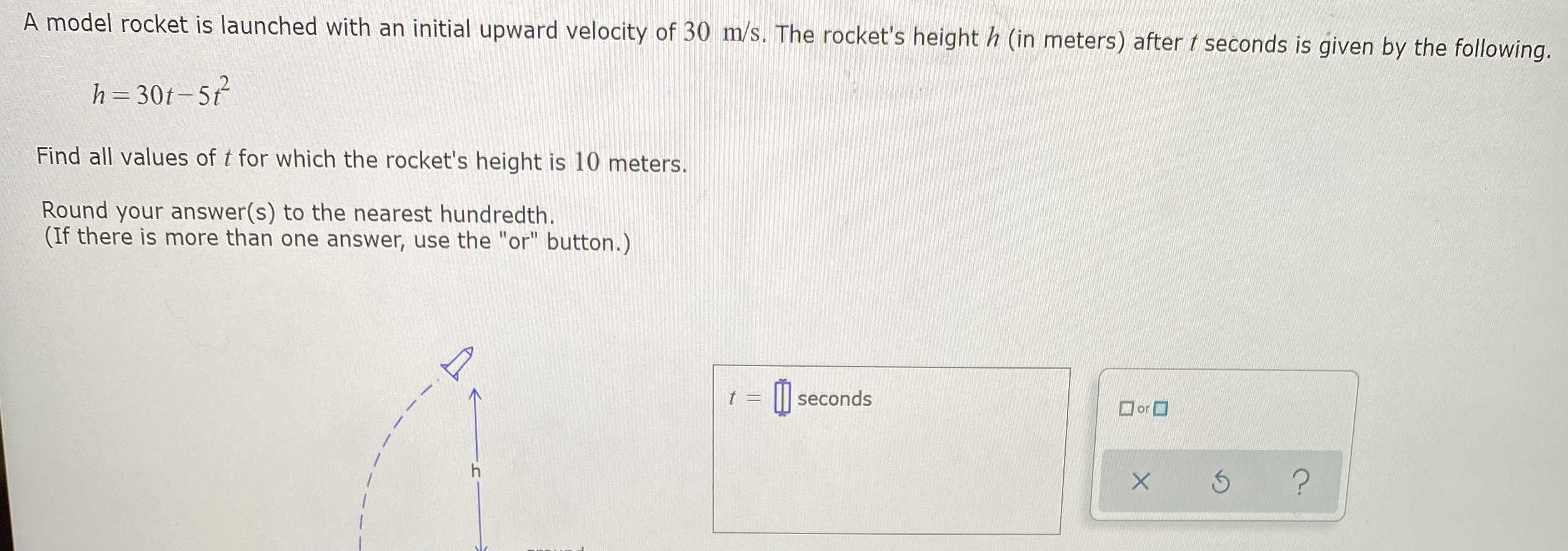### ¿Todavía tienes preguntas de matemáticas?

Pregunte a nuestros tutores expertos
Algebra
PreguntaA model rocket is launched with an initial upward velocity of $$30 m / s$$ . The rocket's height $$h$$ (in meters) after $$t$$ seconds is given by the following.

$$h = 30 t - 5 t ^ { 2 }$$

Find all values of $$t$$ for which the rocket's height is $$10$$ meters.